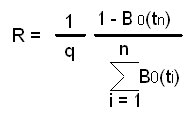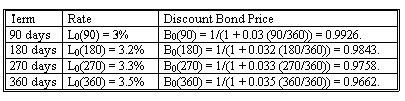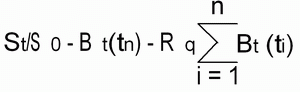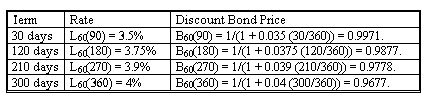### Seeing is believing!

Before you order, simply sign up for a free user account and in seconds you'll be experiencing the best in CFA exam preparation.

### Subject 5. Equity swaps

Characteristics

• One party pays the return on an equity. The other pays fixed, floating, or the return on another equity.
• Rate of return is paid, so payment can be negative.
• Payment is not determined until end of period.

Cash flow to party paying stock and receiving fixedExample 1

IVM enters into a swap with FNS to pay S&P 500 Total Return and receive a fixed rate of 3.45%. The index starts at 2710.55. Payments every 90 days for one year. Net payment will be \$25,000,000 [0.0345 x (90/360) - Return on stock index over settlement period].

After-the-Fact Payments in Equity Swap to Pay S&P 500 Total Return Index and Receive a Fixed Rate of 3.45% (Notional Principal: \$25,000,000)Note: This combination of outcomes on the above dates represents only one of an infinite number of possible outcomes to the swap. They are used to illustrate how the payments are determined and not the likely results.

• The fixed payment will be \$25,000,000 (.0345) (90/360) = \$215,625.
• The first equity payment is \$25,000,000 (2764.90/2710.55 - 1) = \$501,282.
• So the first net payment is IVM pays \$285,657.

If IVM had received floating, the payoff formula would be (Notional principal) x [LIBOR x (days/360) - Return on stock over settlement period].

If the swap were structured so that IVM pays the return on one stock index and receives the return on another, the payoff formula would be (Notional principal) x [Return on one stock index - Return on the other stock index].

Pricing and Valuation of Equity Swaps

For a swap to pay fixed and receive equity, we replicate as follows:

• Invest \$1 in stock.
• Issue \$1 face value loan with interest at rate R. Pay interest on each swap settlement date and repay principal at swap termination date. Interest based on q = days/360.

Example 2

Assume payments on days 180 and 360.

• On day 180, stock worth S180/S0. Sell stock and withdraw S180/S0 - 1.
• Owe interest of Rq.
• Overall cash flow is S180/S0 - 1 - Rq, which is equivalent to the first swap payment. \$1 is left over. Reinvest in the stock.
• On day 360, stock is worth S360/S180.
• Liquidate stock. Pay back loan of \$1 and interest of Rq.
• Overall cash flow is S360/S180 - 1 - Rq, which is equivalent to the second swap payment.

The value of the position is the value of the swap. In general for n payments, the value at the start isSetting the value to zero and solving for R givesThis is precisely the formula for the fixed rate on an interest rate swap or a currency swap.

Continue with IVM's swap:With q = 90/360, the 1/q = 4, and the fixed rate would, therefore, be R = 4 [(1 - 0.9662) / (0.9926 + 0.9843 + 0.9758 + 0.9662)] = 0.0345. Thus, the rate would be 3.45%.

To value the swap at time t during its life, consider the party paying fixed and receiving equity.

• To replicate the first payment, at time t: purchase 1/S0 shares at a cost of (1/S0)St. Borrow \$1 at rate R maturing at next payment date.
• At the next payment date (assume day 90), shares are worth (1/S0)S90. Sell the stock, generating (1/S0) S90 - 1 (equivalent to the equity payment on the swap), plus \$1 left over, which is reinvested in the stock. Pay the loan interest, Rq (which is equivalent to the fixed payment on the swap).
• Do this for each payment on the swap.

The cost to do this strategy at time t isThis is the value of the swap.

Suppose we are now 60 days into the life of the IVM swap. The new term structure is as follows:The stock index is at 2739.60. Thus, the value of the swap per \$1 notional principal is (2739.60/2710.55) - 0.9677 - 0.0345 (90/360) (0.9971 + 0.9877 + 0.9778 + 0.9677) = 0.00911854.

The formulation, however, is from the perspective of the party paying the fixed rate and receiving the equity return. So to IVM, the value is actually -0.00911854 per \$1 notional principal. Thus, for a notional principal of \$25 million, the value of the swap is \$25,000,000 x (-0.00911854) = -\$227,964.

To value the equity swap receiving floating and paying equity, note the equivalence to

• A swap to pay equity and receive fixed, plus
• A swap to pay fixed and receive floating.

So we can use what we already know.

• Using the new discount factors, the value of the fixed payments (plus hypothetical notional principal) is 0.0345 (90/360) (0.9971 + 0.9877 + 0.9778 + 0.9677) + 1(0.9677) = 1.00159884.
• The value of the floating payments (plus hypothetical notional principal) is (1 + .03 (90/360)) (0.9971) = 1.00457825.
• The plain vanilla swap value is, thus, 1.00457825 - 1.00159884 = -0.00297941.
• For a \$25 million notional principal, \$25,000,000(-0.00297941) = -\$74,485.
• So the value of the equity swap is (using -\$227,964, the value of the equity swap to pay fixed): -\$227,964 - \$74,485 = -\$302,449.

For swaps to pay one equity and receive another, replicate by selling short one stock and buy the other. Each period withdraw the cash return, reinvesting \$1. Cover short position by buying it back, and then sell short \$1. So each period start with \$1 long one stock and \$1 short the other.

For the IVM swap, suppose we pay the S&P and receive NASDAQ, which starts at 1835.24 and goes to 1915.71. The value of the swap is (1915.71/1835.24) - (2739.60/2710.55) = 0.03312974. For \$25 million notional principal, the value is \$25,000,000 (0.03312974) = \$828,244.

#### Practice Question 1

A brokerage firm, which has \$10 million in S&P500 stocks, enters into an equity swap with a pension fund which currently has \$10 million in a short term savings account earning LIBOR. If these two entities were to arrange a proper equity swap, in which payments are to be made annually, what would be the net payment for a period that saw the S&P500 net 12% and LIBOR set at 8%?

A. Brokerage firm makes a net payment of \$400,000.
B. Pension plan makes a net payment of \$400,000.
C. Pension plan makes a net payment of \$200,000.